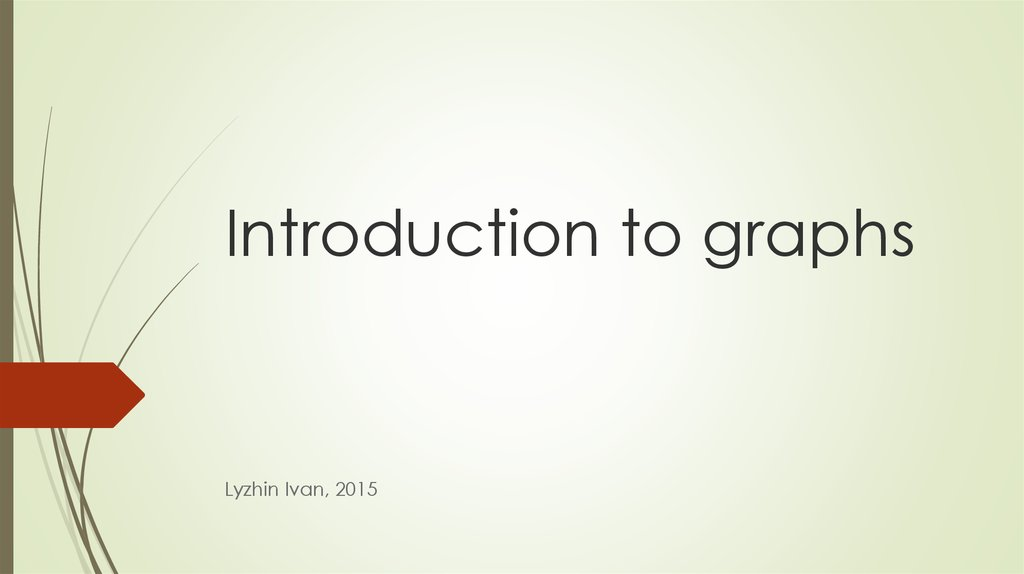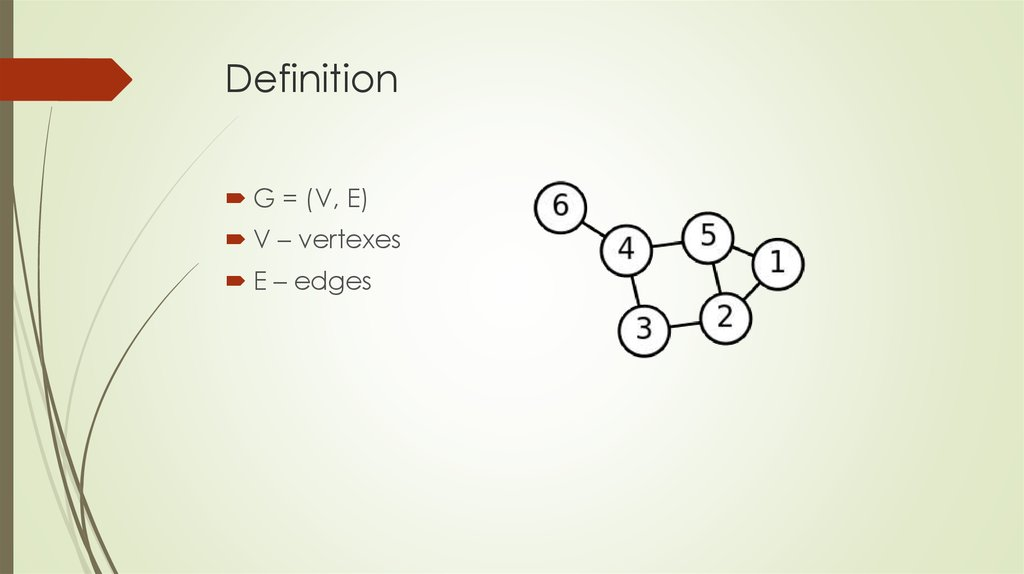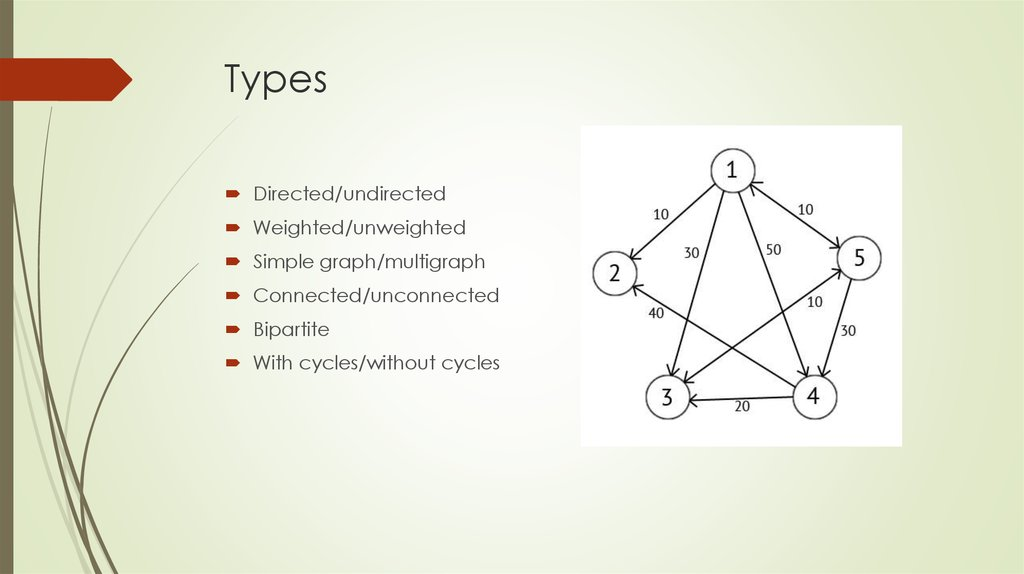# Introduction to graphs

## 1. Introduction to graphs

Lyzhin Ivan, 2015

G = (V, E)
V – vertexes
E – edges

## 3. Types

Directed/undirected
Weighted/unweighted
Simple graph/multigraph
Connected/unconnected
Bipartite
With cycles/without cycles

1
2
3
4
5
1
0
10
30
50
10
2
0
0
0
0
0
3
0
0
0
0
10
4
0
40
20
0
0
5
10
0
10
30
0
Memory: O(|V|^2)

## 5. Ways of presenting in memory Incidence matrix

1
2
3
4
5
6
7
1
0
0
1
0
0
0
1
2
0
0
0
0
1
1
1
3
0
0
0
1
0
1
0
4
1
1
0
1
0
0
0
5
0
1
1
0
1
0
0
6
1
0
0
0
0
0
0
Memory: O(|V|*|E|)

u
v
w
1
2
10
1
3
30
1
4
50
1
5
10
3
5
10
4
2
40
4
3
20
5
1
10
5
3
10
5
4
30
Memory: O(|E|)

1
(2, 10)
(3, 30)
(5, 50)
2
3
(5, 10)
4
(2, 40)
(3, 20)
5
(1, 10)
(3, 10)
Memory: O(|E|)
(4, 30)
(5, 10)

## 8. Problems without explicit graph

Labyrinth
Number of objects

## 9. Basic algorithms Depth-First Search (DFS)

void dfs(int u)
{
if (used[u]) return;
used[u] = true;
for (auto v : g[u])
dfs(v);
}
Complexity: O(|V|+|E|)

## 10. Basic algorithms Breadth-First Search (BFS)

void bfs(int s)
{
queue<int> q;
q.push(s);
used[s] = true;
while(!q.empty())
{
int u = q.front();
q.pop();
for(auto v: g[u])
if(!used[v])
{
q.push(v);
used[v] = true;
}
}
}
Complexity: O(|V|+|E|)

## 11. Examples

Find cycle in graph
Count number of connected components in graph
Find distance and path from one vertex to each other in unweighted graph